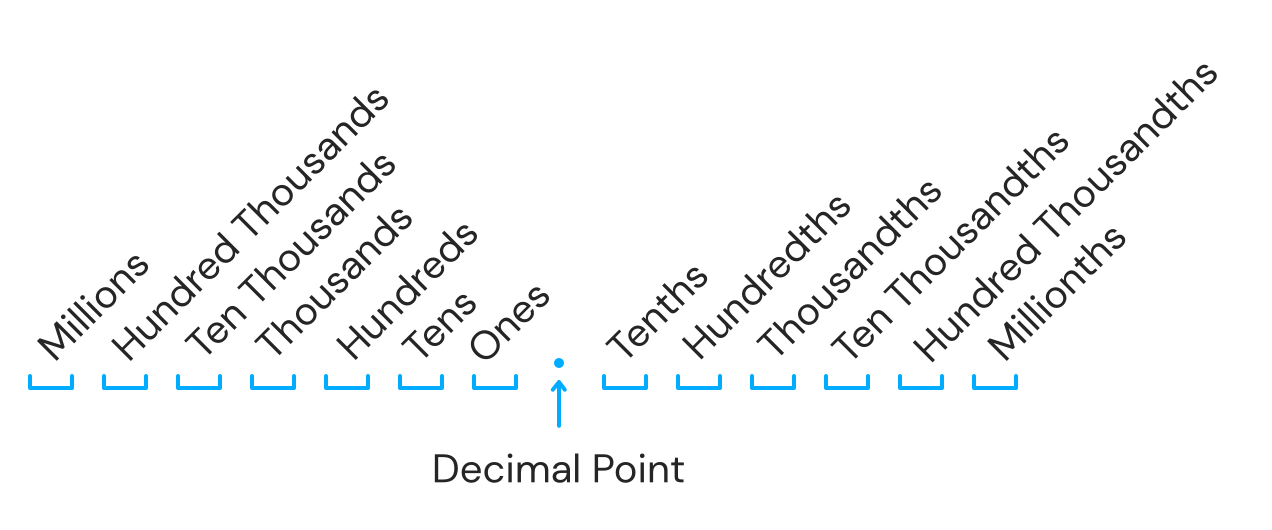### Still have math questions?

Scientific Notation Length Conversion Area Conversion

### Calculator

Percent
%
Decimal
Fraction

#### Knowledge

##### Percent

Percentage (from Latin per centum "by a hundred") is a number or ratio expressed as a fraction of 100.

A percent is a representation of a number as a part of one hundred. The word "percent" can be written "per cent" which means "per 100" or "/100".

We use the symbol (%) to denote a percentage:

N% = N 100

##### Fraction

A fraction is a real number written as a quotient, or ratio, of two integers a and b, where b ≠ 0.The integer above the fraction bar is called the numerator and the integer below is called the denominator. The numerator is often called the "part" and the denominator is often called the "whole." Equivalent fractions are two equal ratios expressed using different numerators and denominators. For example,

50100 = 12

##### Decimal

In this section, we provide a brief review of the decimal system. A real number in decimal form, a decimal consists of a decimal point, digits (0 through 9) to the left of the decimal point representing the whole number part, and digits to the right of the decimal point representing the fractional part. The digits represent powers of 10 as shown in the set {…, 1,000, 100, 10, 1, 1/10, 1/100, 1/1,000, …} according to the following diagram:For example, the decimal 538.3 can be written in the following expanded form:

5 × 100 + 3 × 10 + 8 × 1 + 3 × 110

After simplifying, we obtain the mixed number 538310 . Use this process to convert decimals to mixed numbers.

#### Example 1

30% of 60 is what number?

30100 = n60   Solve for n.

100n = 1800

n = 18

#### Example 2

105300 = 105÷15300÷15 = 720

#### Example 3

Write as a decimal: 3/4.

Use long division to convert to a decimal.Please provide numbers only. Please provide at least two numbers. Please provide 2 to 10 non-zero integer numbers. None